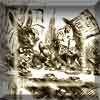#### You may also like### A Problem of Time

Consider a watch face which has identical hands and identical marks for the hours. It is opposite to a mirror. When is the time as read direct and in the mirror exactly the same between 6 and 7?### Fit for Photocopying

Explore the relationships between different paper sizes.### Coloured Black

An ink stamp draws out a shape when it is rotated. What is its area?

# Back in Time

##### Age 14 to 16 ShortChallenge Level

The only digits which will appear the same when reflected in the glass table-top are $0, 1, 3,$ and $8$. So it it necessary to find the number of times in a $24$-hour period that the display on the clock is made up only of these digits.

There are two possibilities for the first digit: $0$ or $1$.
There are four possibilities for the second digit: $0$, $1$, $3$ or $8$.
There are three possibilities for the third digit: $0$, $1$ or $3$.
There are four possibilities for the fourth digit: $0$, $1$, $3$ or $8$.

To find the total number of possible times, we can multiply together the number of possibilities for each digit.

Therefore the display and its reflection give the same time on
$2 \times 4 \times 3 \times 4 =96$ occasions

This problem is taken from the UKMT Mathematical Challenges.
You can find more short problems, arranged by curriculum topic, in our short problems collection.